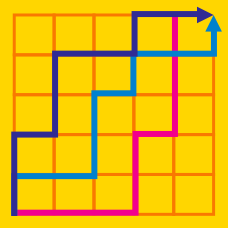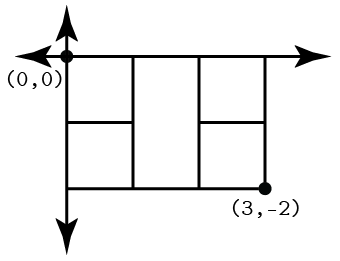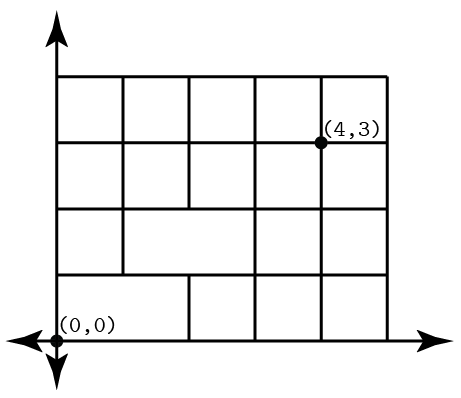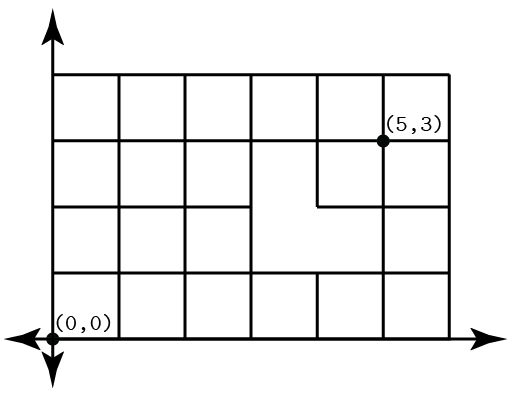Probability

# Rectangular Grid Walk - Walls

An ant crawling along the coordinate plane wishes to travel from the origin to $(3, \, -2)$ while avoiding a wall between $(1, \, -1)$ and $(2, \, -1)$. If each move the ant makes must be $1$ unit right or down, then how many possible paths could the ant take?In a game of basketball, the underdog's advantage says that whenever one team has one more point than the other, the losing team will score a point. Given that teams score one point at a time in this basketball game until one team has $8$ points, in how many different ways could the game unfold?

Pablo is at the point $(4, \, 3)$ in the coordinate plane and is able to walk $1$ unit left or down each step he takes. There is a wall between $(2, \, 1)$ and $(2, \, 2)$ and between $(1, 0)$ and $(1, \, 1)$. How many possible paths could Pablo take from $(4, \, 3)$ to the origin?In a game of soccer, the home team advantage says that whenever the home team is one point away from winning, the other team is unable to score. Given that teams score one point at a time in a soccer game until one team has $5$ points, in how many different ways could the game unfold?

Carmen San Diego is hiding in the coordinate plane at $(5, \, 3)$. She has a trap set between $(3, \, 2)$ and $(4, \, 2)$ and between $(4, \, 1)$ and $(4, \, 2)$. If you can start at the origin and can only move $1$ unit right or up each turn, in how many ways can you get to her position?×